SLVSAQ5E March   2012  – May 2017

PRODUCTION DATA.

1. Features
2. Applications
3. Description
4. Revision History
5. Pin Configuration and Functions
6. Specifications
7. Detailed Description
1. 7.1 Overview
2. 7.2 Functional Block Diagram
3. 7.3 Feature Description
4. 7.4 Device Functional Modes
8. Application and Implementation
1. 8.1 Application Information
2. 8.2 Typical Application
3. 8.3 System Examples
9. Power Supply Recommendations
10. 10Layout
11. 11Device and Documentation Support
12. 12Mechanical, Packaging, and Orderable Information

• DSG|8
• DSG|8

## 8 Application and Implementation

NOTE

Information in the following applications sections is not part of the TI component specification, and TI does not warrant its accuracy or completeness. TI’s customers are responsible for determining suitability of components for their purposes. Customers should validate and test their design implementation to confirm system functionality.

### 8.1 Application Information

The TPS62125 is a high-efficiency synchronous step-down converter providing a wide output voltage range from 1.2 V to 10 V.

### 8.2 Typical Application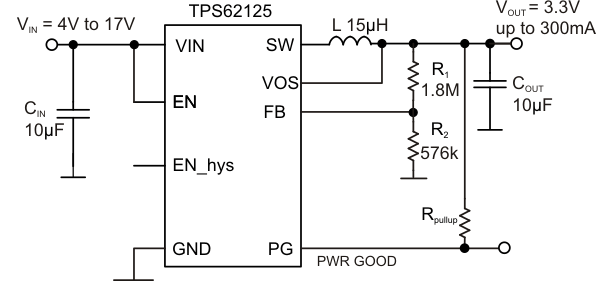Figure 8. TPS62125 3.3-V Output Voltage Configuration

#### 8.2.1 Design Requirements

The device operates over an input voltage range from 3 V to 17 V. The output voltage is adjustable using an external feedback divider.

#### 8.2.2.1 Output Voltage Setting

The output voltage can be calculated by:

Equation 5.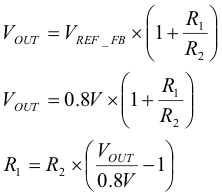The internal reference voltage for the error amplifier, VREF_FB, is nominal 0.808 V. However for the feedback resistor divider selection, it is recommended to use the value 0.800 V as the reference. Using this value, the output voltage sets 1% higher and provides more headroom for load transients as well for line and load regulation. The current through the feedback resistors R1 and R2 should be higher than 1 µA. In applications operating over full temperature range or in noisy environments, this current may be increased for robust operation. However, higher currents through the feedback resistors impact the light load efficiency of the converter.

Table 1 shows a selection of suggested values for the feedback divider network for most common output voltages.

### Table 1. Suggested Values for Feedback Divider Network

OUTPUT VOLTAGE 1.2 V 1.8 V 3.3 V 5 V 6.7 V 8 V
R1 [kΩ] 180 300 1800 1100 1475 1800
R2 [kΩ] 360 240 576 210 200 200

#### 8.2.2.2 Enable Threshold and Hysteresis Setting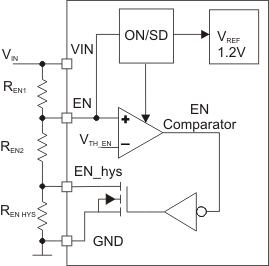Figure 9. Using the Enable Comparator Threshold and Hysteresis for an Input SVS (Supply Voltage Supervisor)

The enable comparator can be used as an adjustable input supply voltage supervisor (SVS) to start and stop the DC/DC converter depending on the input voltage level. The input voltage level, VIN_startup, at which the device starts up is set by the resistors REN1 and REN2 and can be calculated by :

Equation 6.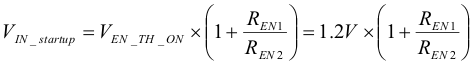The resistor values REN1 and REN2 can be calculated by:

Equation 7.Equation 8.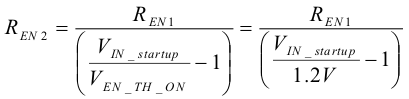The input voltage level VIN_stop at which the device will stop operation is set by REN1, REN2 and REN HYS and can be calculated by:

Equation 9.The resistor value REN_hys can be calculated according to:

Equation 10.The current through the resistors REN1, REN2, and REN HYS should be higher than 1 µA. In applications operating over the full temperature range and in noisy environments, the resistor values can be reduced to smaller values.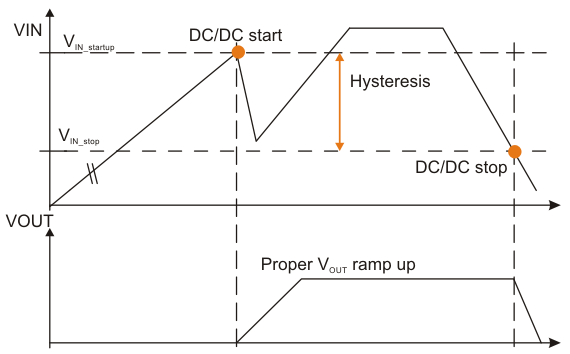Figure 10. Using the EN Comparator as Input SVS for Proper VOUT Ramp Up

#### 8.2.2.3 Power Good (PG) Pullup and Output Discharge Resistor

The power good open collector output needs an external pull up resistor to indicate a high level. The pull up resistor can be connected to a voltage level up to 10 V. The output can sink current up to 0.4 mA with specified output low level of less than 0.3 V. The lowest value for the pull up resistor can be calculated by:

Equation 11.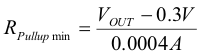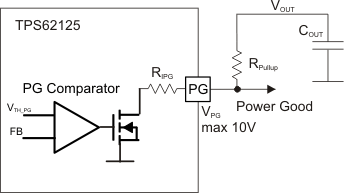Figure 11. PG Open Collector Output

The PG pin can be used to discharge the output capacitor. The PG output has an internal resistance RIPG of typical 600 Ω and minimum 400 Ω. The maximum sink current into the PG pin is 10 mA. In order to limit the discharge current to the maximum allowable sink current into the PG pin, the external pull up resistor RPull up can be calculated to:

Equation 12.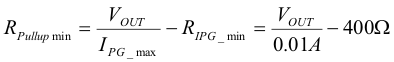In case a negative value is calculated, the external pull up resistor can be removed and the PG pin can be directly connected to the output.

#### 8.2.2.4 Output Filter Design (Inductor and Output Capacitor)

The external components have to fulfill the needs of the application, but also the stability criteria of the devices control loop. The TPS62125 is optimized to work within a range of L and C combinations. The LC output filter inductance and capacitance have to be considered together, creating a double pole, responsible for the corner frequency of the converter. Table 2 can be used to simplify the output filter component selection.

### Table 2. Recommended LC Output Filter Combinations

INDUCTOR VALUE [µH](2) OUTPUT CAPACITOR VALUE [µF](1)
10 µF 2 x 10 µF 22 µF 47 µF
VOUT 1.2 V - 1.8 V
15
22 (3)
VOUT 1.8 V - 3.3 V
15 (3)
22 (3)
VOUT 3.3 V - 5 V
10
15 (3) (3)
22
VOUT 5 V - 10 V
10 (3) (3)
15
22
Capacitance tolerance and bias voltage de-rating is anticipated. The effective capacitance can vary by 20% and -50%.
Inductor tolerance and current de-rating is anticipated. The effective inductance can vary by 20% and -30%.
This LC combination is the standard value and recommended for most applications.

More detailed information on further LC combinations can be found in application note SLVA515.

#### 8.2.2.5 Inductor Selection

The inductor value affects its peak-to-peak ripple current, the PWM-to-PFM transition point, the output voltage ripple and the efficiency. The selected inductor has to be rated for its DC resistance and saturation current. The inductor ripple current (ΔIL) decreases with higher inductance and increases with higher VIN or VOUT and can be estimated according to Equation 13.

Equation 14 calculates the maximum inductor current under static load conditions. The saturation current of the inductor should be rated higher than the maximum inductor current as calculated with Equation 14. This is recommended because during heavy load transient the inductor current will rise above the calculated value. A more conservative way is to select the inductor saturation current according to the high-side MOSFET switch current limit ILIMF.

Equation 13.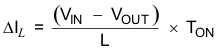Equation 14.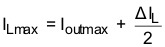where

• TON: See Equation 1
• L: Inductance
• ΔIL: Peak to Peak inductor ripple current
• ILmax: Maximum Inductor current

In DC/DC converter applications, the efficiency is essentially affected by the inductor AC resistance (i.e. quality factor) and by the inductor DCR value. To achieve high-efficiency operation, take care in selecting inductors featuring a quality factor above 25 at the switching frequency. Increasing the inductor value produces lower RMS currents, but degrades transient response. For a given physical inductor size, increased inductance usually results in an inductor with lower saturation current.

The total losses of the coil consist of both the losses in the DC resistance (RDC) and the following frequency-dependent components:

• The losses in the core material (magnetic hysteresis loss, especially at high switching frequencies)
• Additional losses in the conductor from the skin effect (current displacement at high frequencies)
• Magnetic field losses of the neighboring windings (proximity effect)

The following inductor series from different suppliers have been used with the TPS62125.

### Table 3. List of Inductors

INDUCTANCE [µH] DCR [Ω] DIMENSIONS [mm3] INDUCTOR TYPE SUPPLIER
10 / 15 0.33 max / 0.44 max. 3.3 x 3.3 x 1.4 LPS3314 Coilcraft
22 0.36 max. 3.9 x 3.9 x 1.8 LPS4018 Coilcraft
15 0.33 max. 3.0 x 2.5 x 1.5 VLF302515 TDK
10/15 0.44 max / 0.7 max. 3.0 x 3.0 x 1.5 LPS3015 Coilcraft
10 0.38 typ. 3.2 × 2.5 × 1.7 LQH32PN Murata

#### 8.2.2.6 Output Capacitor Selection

Ceramic capacitors with low ESR values provide the lowest output voltage ripple and are recommended. The output capacitor requires either an X7R or X5R dielectric. Y5V and Z5U dielectric capacitors, aside from their wide variation in capacitance over temperature, become resistive at high frequencies.

At light load currents the converter operates in power-save mode and the output voltage ripple is dependent on the output capacitor value and the PFM peak inductor current. Higher output capacitor values minimize the voltage ripple in PFM Mode and tighten DC output accuracy in PFM mode. In order to achieve specified regulation performance and low-output voltage ripple, the DC-bias characteristic of ceramic capacitors must be considered. The effective capacitance of ceramic capacitors drops with increasing DC-bias voltage. Due to this effect, it is recommended for output voltages above 3.3 V to use at least 1 x 22-µF or 2 x 10-µF ceramic capacitors on the output.

#### 8.2.2.7 Input Capacitor Selection

Because of the nature of the buck converter having a pulsating input current, a low ESR input capacitor is required for best input voltage filtering and minimizing the interference with other circuits caused by high input voltage spikes. For most applications, a 10-µF ceramic capacitor is recommended. The voltage rating and DC bias characteristic of ceramic capacitors need to be considered. The input capacitor can be increased without any limit for better input voltage filtering.

For applications powered from high impedance sources, a tantalum polymer capacitor should be used to buffer the input voltage for the TPS62125. Tantalum polymer capacitors provide a constant capacitance vs. DC bias characteristic compared to ceramic capacitors. In this case, a 10-µF ceramic capacitor should be used in parallel to the tantalum polymer capacitor to provide low ESR.

Take care when using only small ceramic input capacitors. When a ceramic capacitor is used at the input and the power is being supplied through long wires, such as from a wall adapter, a load step at the output or VIN step on the input can induce large ringing at the VIN pin. This ringing can couple to the output and be mistaken as loop instability or could even damage the part by exceeding the maximum ratings. In case the power is supplied via a connector e.g. from a wall adapter, a hot-plug event can cause voltage overshoots on the VIN pin exceeding the absolute maximum ratings and can damage the device, too. In this case a tantalum polymer capacitor or overvoltage protection circuit reduces the voltage overshoot, see Figure 45.

Table 4 shows a list of input/output capacitors.

### Table 4. List of Capacitors

CAPACITANCE [µF] SIZE CAPACITOR TYPE USAGE SUPPLIER
10 0805 GRM21B 25V X5R CIN /COUT Murata
10 0805 GRM21B 16V X5R COUT Murata
22 1206 GRM31CR61 16V X5R COUT Murata
22 B2 (3.5x2.8x1.9) 20TQC22MYFB CIN / input protection Sanyo

.

#### 8.2.3 Application Curves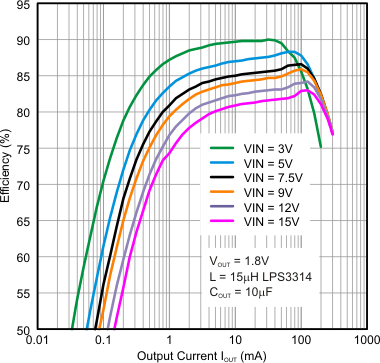Figure 12. Efficiency vs. Output Current VOUT = 1.8 V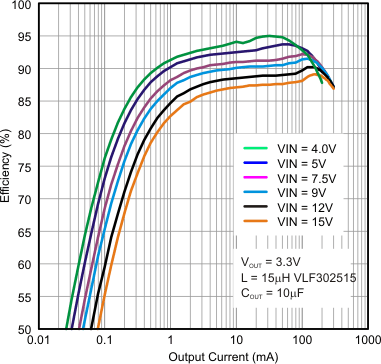Figure 14. Efficiency vs. Output current, VOUT = 3.3 VFigure 16. Efficiency vs. Output Current, VOUT = 5 VFigure 18. Efficiency vs. Output current, VOUT = 6.8 VFigure 20. Efficiency vs. Output Current, VOUT = 8 V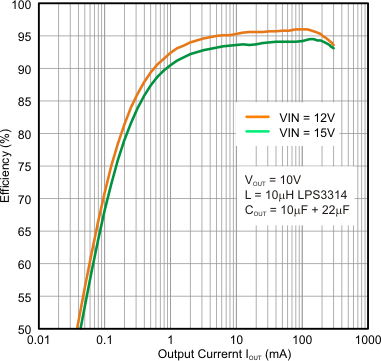Figure 22. Efficiency vs. Output Current, VOUT = 10 V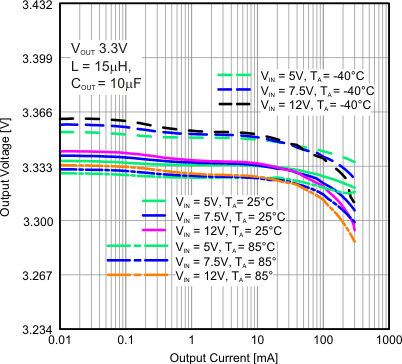Figure 24. Output Voltage vs. Output Current, VOUT = 3.3 V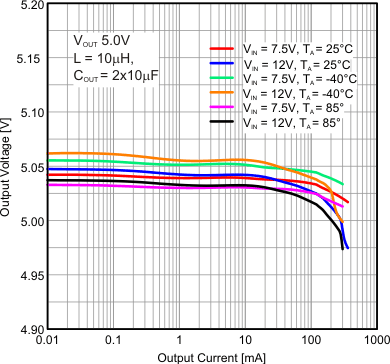Figure 26. Output Voltage vs. Output current, VOUT = 5 V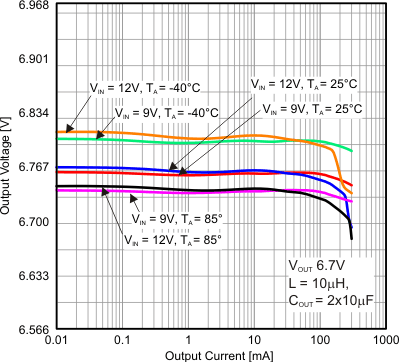Figure 28. Output Voltage vs. Output Current, VOUT = 6.7 V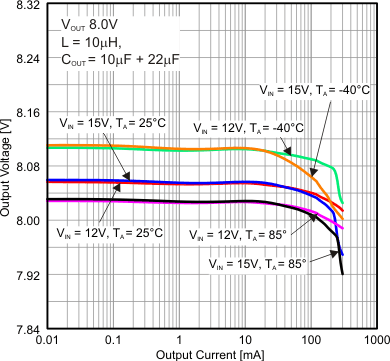Figure 30. Output Voltage vs. Output Current, VOUT = 8 V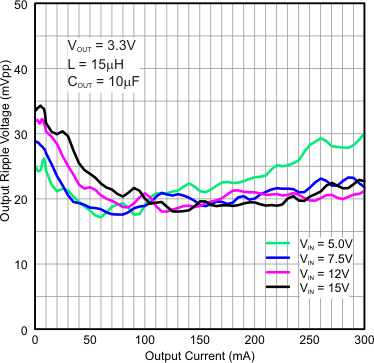Figure 32. Output Ripple Voltage vs. Output Current,
VOUT = 3.3 VFigure 34. Switch Frequency vs. Output Current, VOUT 5 V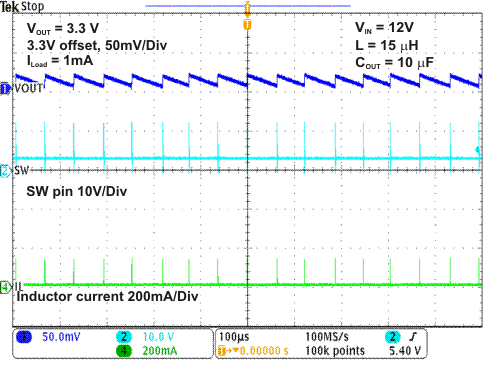Figure 36. Power-Save Mode VOUT= 3.3 V, IOUT = 1 mAFigure 38. Load Transient 5 mA to 200 mA, VOUT = 3.3 V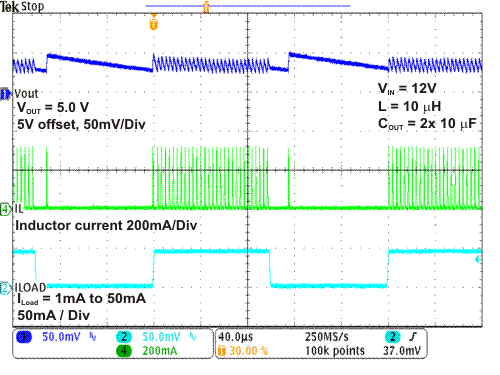Figure 40. Load Transient 1 mA to 50 mA, VOUT = 5 VFigure 42. AC Load Regulation VOUT = 5 V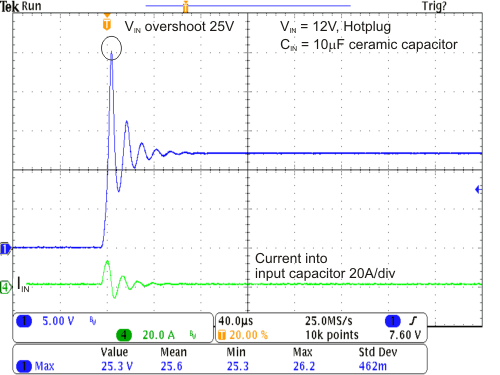Figure 44. VIN Hotplug Overshoot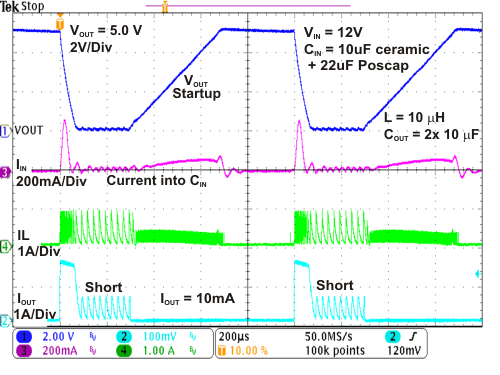Figure 46. Short Circuit and Overcurrent ProtectionFigure 48. Operation With EN = VIN, VIN Tracks VOUT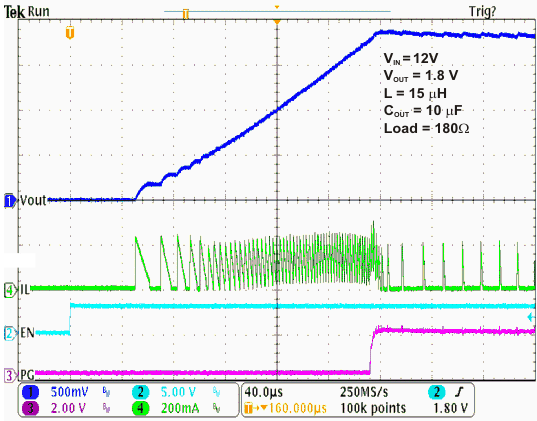Figure 50. Start-Up 1.8 V VOUT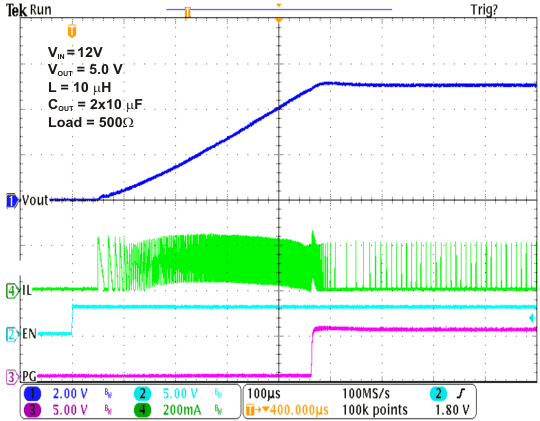Figure 52. Start-Up 5 V VOUT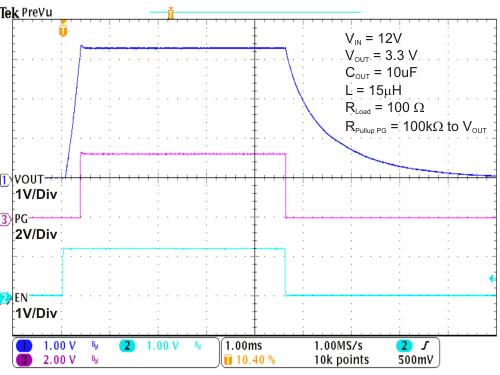Figure 54. VOUT Ramp Up/Down With EN On/Off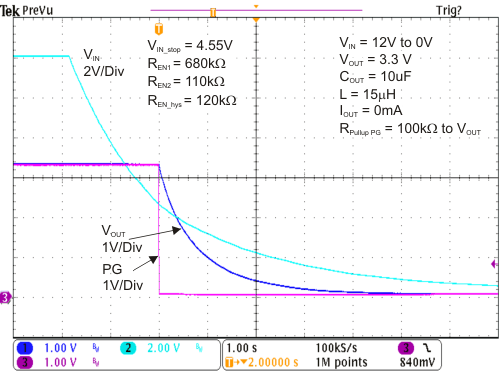Figure 56. VOUT Ramp Down With Falling VIN, See Schematic Figure 59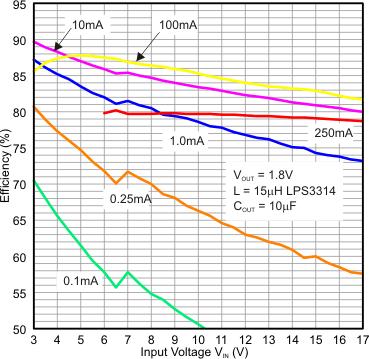Figure 13. Efficiency vs. Input Voltage, VOUT = 1.8 V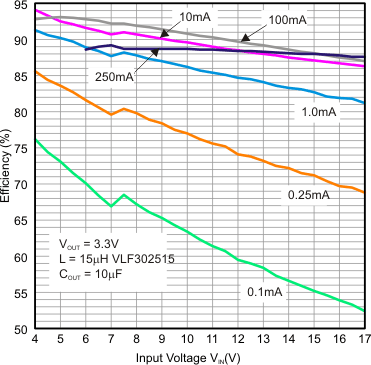Figure 15. Efficiency vs. Input voltage, VOUT = 3.3 V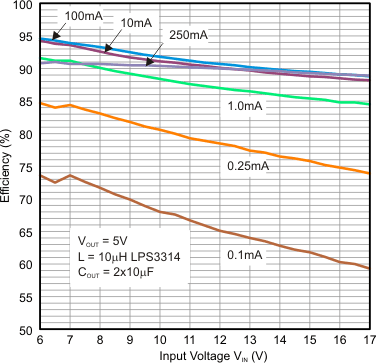Figure 17. Efficiency vs. Input Voltage, VOUT = 5 V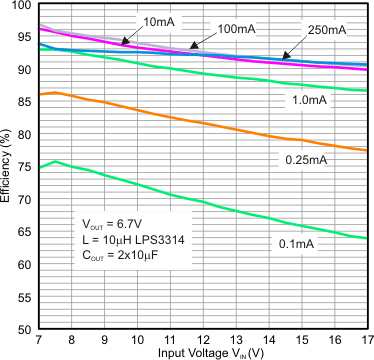Figure 19. Efficiency vs. Input Voltage, VOUT = 6.8 V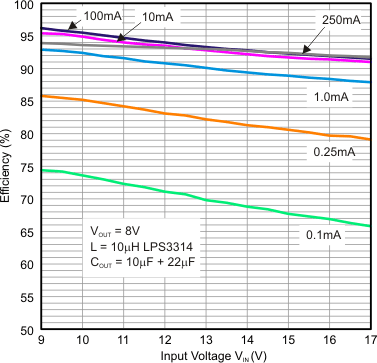Figure 21. Efficiency vs. Input Voltage, VOUT = 8 V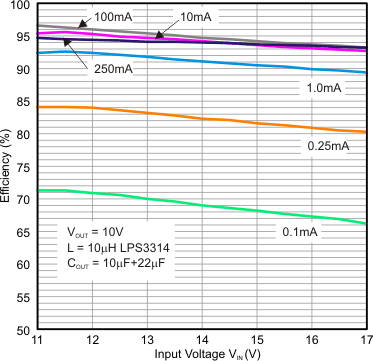Figure 23. Efficiency vs. Input Voltage, VOUT = 10 V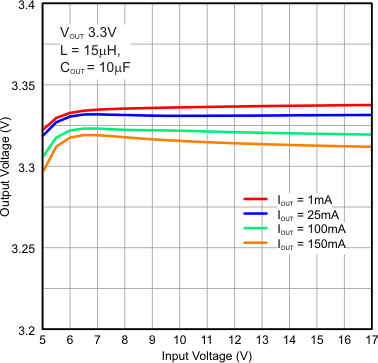Figure 25. Output Voltage vs. Input Voltage, VOUT = 3.3 V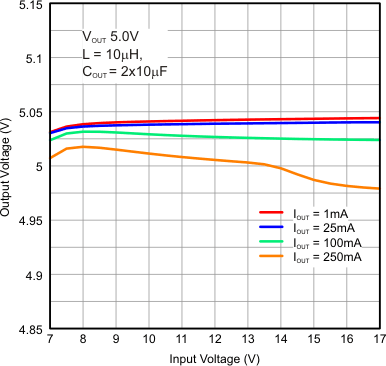Figure 27. Output Voltage vs. Input Voltage, VOUT = 5 V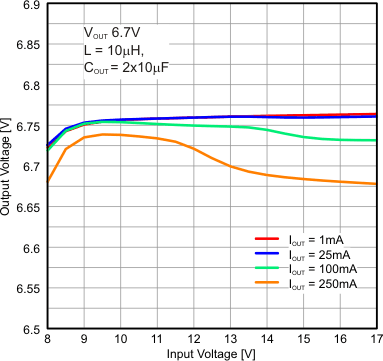Figure 29. Output voltage vs. Input voltage, VOUT = 6.7 VFigure 31. Output Voltage vs. Input Voltage, VOUT = 8 VFigure 33. Switch Frequency vs. Output Current,
VOUT = 3.3 V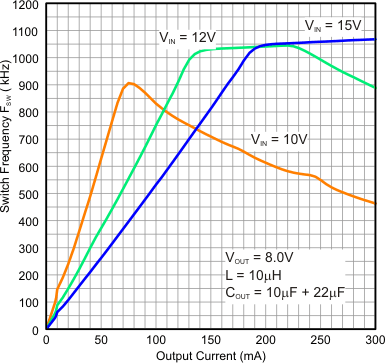Figure 35. Switch Frequency vs. Output Current, VOUT = 8 VFigure 37. PWM Mode VOUT= 3.3 V, IOUT = 100 mA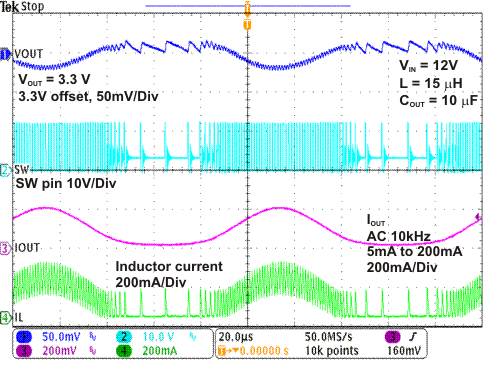Figure 39. AC Load Regulation, VOUT = 3.3 VFigure 41. Load Transient 10 mA to 200 mA, VOUT = 5 V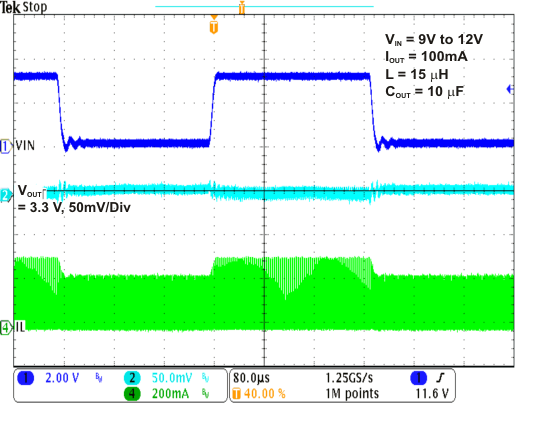Figure 43. Line Transient Response VIN = 9 V to 12 V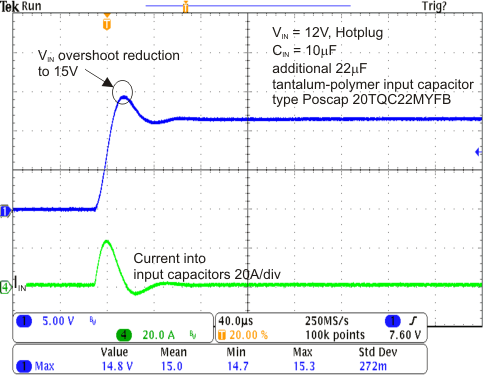Figure 45. VIN Hotplug Overshoot Reduction With Poscap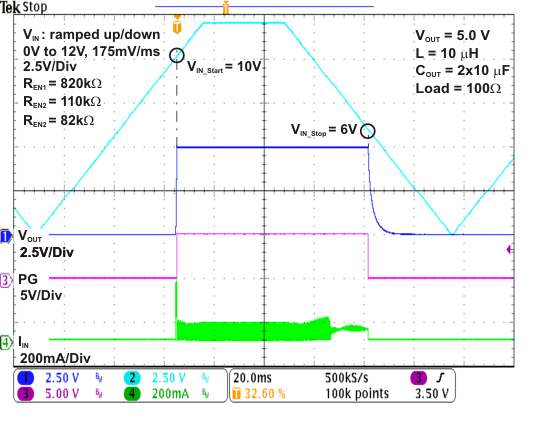Figure 47. Input Supply Voltage Supervisor (SVS),
VOUT = 5 VFigure 49. 0.5 mA Current Source, 20 mA Pulse Load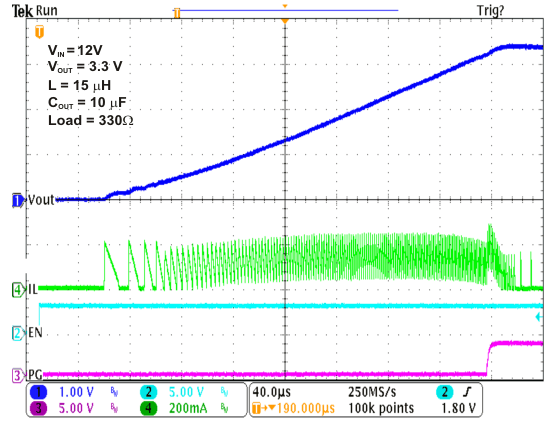Figure 51. Start-Up 3.3 V VOUT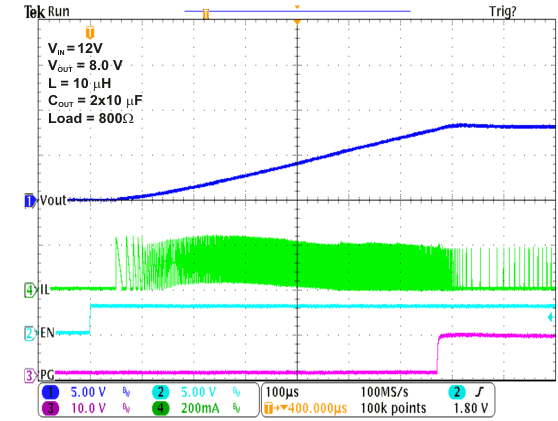Figure 53. Start-Up 8 V VOUT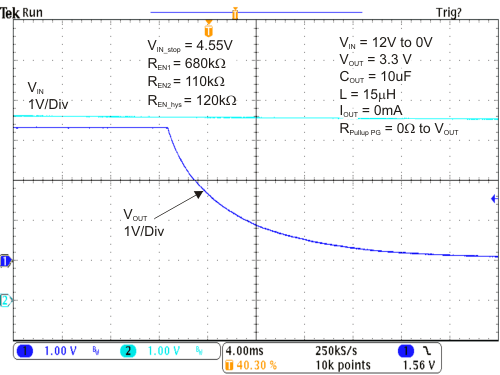Figure 55. Output Discharge Using PG Pin, Triggered by EN Comparator

### 8.3 System Examples

#### 8.3.1 TPS62125 5-V Output Voltage Configuration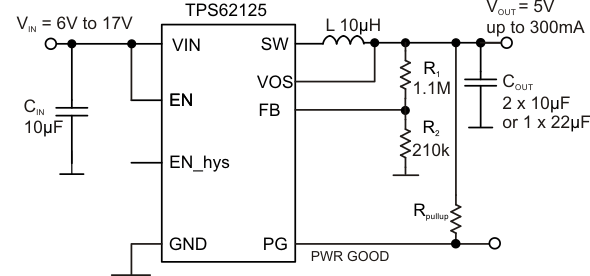Figure 57. TPS62125 5-V Output Voltage Configuration

#### 8.3.2 TPS62125 5-V VOUT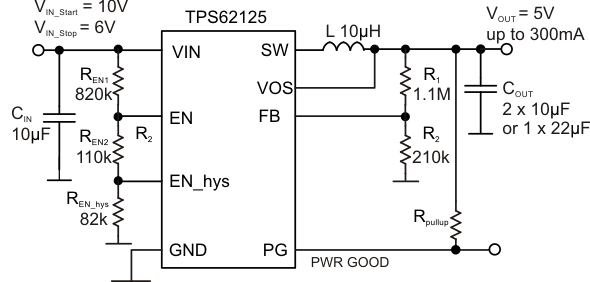Figure 58. TPS62125 5-V VOUT, Start-up Voltage VIN_Start = 10 V, Stop Voltage VIN_Stop = 6 V, See Figure 47

#### 8.3.3 TPS62125 Operation From a Storage Capacitor Charged From a 0.5 mA Current Source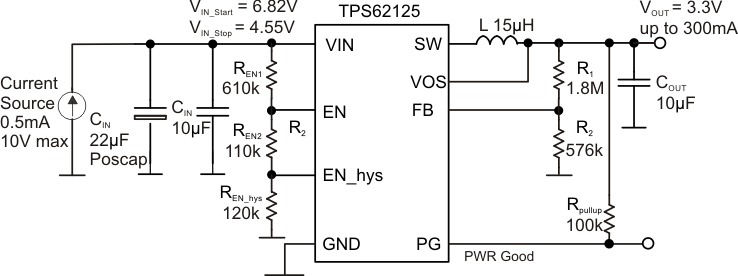Figure 59. TPS62125 Operation From a Storage Capacitor Charged From a 0.5 mA Current Source,
VOUT = 3.3 V, See Figure 49

#### 8.3.4 5 V to –5 V Inverter Configuration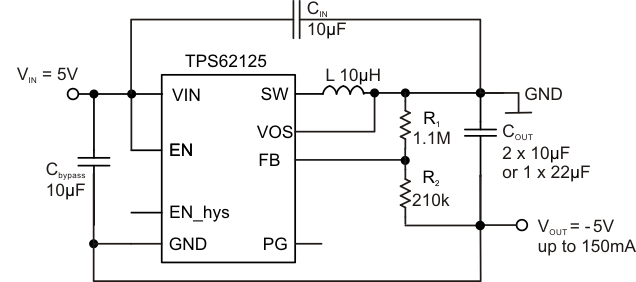Figure 60. 5 V to –5 V Inverter Configuration, See SLVA514